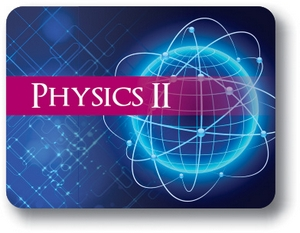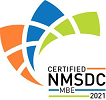Red Comet## Physics - Semester - 2 (LAB)

#### Course Price: \$285.00## Course Details:

This course builds on the fundamentals of physics laid in the first semester. Students learn about the different types of waves and wave properties. They learn about Electromagnetic waves and the dual nature of light. The course discusses energy and the different types of energy and delves into the laws of conservation. Students learn about the fundamental forces – including the Strong force, the electromagnetic force and the Weak force. The course exposes the student to the fundamentals of electricity and the nature of both the magnetic and electric field. The nature of thermal energy is discussed followed by the fundamentals of thermodynamics. Last but not least, there is an extensive discussion on earth’s energy sources – including the various forms of alternate energies available to us.

Note: This course is not designed for ELL (English Language Learners) students. ELL students may enroll in this course ONLY if they have adequate mentor support at their home school and are able to fulfill all course requirements.

## Syllabus:

Section 1: Waves

• Introduction to Waves
• Types of Waves
• Wave Behavior
• Applications of Waves

Section 2: Energy and Work

• Introduction to Work and Power
• Energy and its Types
• Work Energy Relation
• Conservative & Non-Conservative Forces
• Conservation of Mass, Energy and Momentum

Section 3: Electrons, Electricity and Electromagnetism

• Introduction to Electric Field
• Electric Circuits
• Electromagnetic Field
• Generating Electricity

Section 4: Fundamental Forces, Radioactivity & Photoelectric Effect

• Fundamental Force – Electrostatic (Strong) Force
• Photoelectric Effect
• Case Study – Solar cells
• Atomic Model & Mass-Energy Relation
• Trophic Fundamental Force – Weak Nuclear Force

Section 5: Thermodynamics

• Thermal Energy, Heat and Temperature
• Transfer of Thermal Energy
• Introduction to Thermodynamics
• Laws of Thermodynamics
• Case Study – Space Shuttle / Rocket Launch

Section 6: Earth’s Energy Sources

• Introduction to Fossil Fuels
• Case Study – Risks Associated with Fossil Fuel Extraction
• Introduction to Nuclear Energy
• Case Study – Risks Associated with Nuclear Energy
• Introduction to Alternative Energy

### Accreditation & Approvals